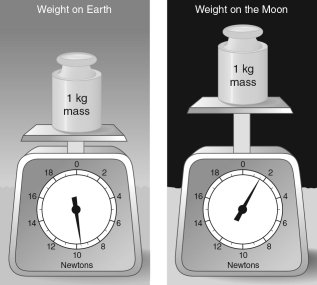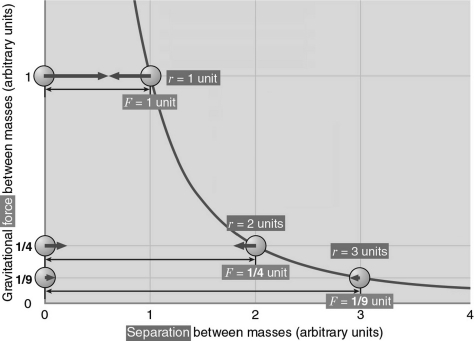# Astronomy The Solar System

Physics & Astronomy

## Quiz 4 : Gravity and OrbitsStudy FlashcardsLooking for Introductory Astronomy Homework Help?

## Quiz 4 :Gravity and Orbits

Question TypeThe force of gravity between Saturn and the Sun is ________ the force of gravity between Earth and the Sun.For reference, Saturn is approximately 100 times more massive than Earth, and the semimajor axis of Saturn's orbit is 10 AU.
Free
Multiple Choice

E

Tags
Choose question tagThe distance used in Newton's law of gravitation is
Free
Multiple Choice

B

Tags
Choose question tagIf you weighed 150 lb on Earth, what would you weigh on Mars? For reference, Mars has a mass that is 0.1 times Earth's mass and Mars has a radius that is 0.5 times Earth's radius.
Free
Multiple Choice

D

Tags
Choose question tagWhich of the following properties of an astronaut changes when he or she is standing on the Moon, relative to when the astronaut is standing on Earth?
Multiple Choice
Tags
Choose question tagSuppose you are suddenly transported to a planet with 1/4 the mass of Earth but the same radius as Earth.Your weight would ________ by a factor of ________.
Multiple Choice
Tags
Choose question tagIf a person having mass, M, is standing on the ground and experiencing an acceleration due to gravity of g, what is the correct formula for the person's weight, F?
Multiple Choice
Tags
Choose question tagThe force of gravity that an object has is directly proportional to its
Multiple Choice
Tags
Choose question tagAn astronaut who weighs 700 N on Earth is in empty space, very far from any other objects.Approximately what is the mass of the astronaut?
Multiple Choice
Tags
Choose question tagTwo rocks (call them S and T) are a distance of 50 km from one another in empty space.Rock S has 20 times the mass of rock T.Considering only their mutual gravitational force, which rock will accelerate faster in response to gravity?
Multiple Choice
Tags
Choose question tagTwo rocks (call them S and T) are released at the same time from the same height and start from rest.Rock S has 20 times the mass of rock T.Which rock will fall faster if the only forces involved are each rock's mutual gravitational attraction with Earth?
Multiple Choice
Tags
Choose question tagIf the distance between Earth and the Sun were cut in half, the gravitational force between these two objects would
Multiple Choice
Tags
Choose question tagSuppose you are suddenly transported to a planet that had 1/4 the radius of Earth but the same mass as Earth.Your weight would ________ by a factor of ________.
Multiple Choice
Tags
Choose question tagA quantity that follows an inverse square law
Multiple Choice
Tags
Choose question tagEarth is held together in the shape of a sphere.This is due to
Multiple Choice
Tags
Choose question tagBetween any two objects with mass, the force of gravity is always
Multiple Choice
Tags
Choose question tagThe force of gravity between Earth and the Sun is approximately ________ the force of gravity between Earth and the Moon.For reference, the average distance between Earth and the Moon is 0.003 astronomical unit (AU), the mass of the Moon is 7 1022 kg, and the mass of the Sun is 2 1030 kg.
Multiple Choice
Tags
Choose question tagIn the absence of air friction, a 0.001-kg piece of paper and a 0.1-kg notebook are dropped from the same height and allowed to fall to the ground.How do their accelerations compare?
Multiple Choice
Tags
Choose question tagAccording to the scales shown in the figure below, about how many times stronger is gravity on Earth than on the Moon?Multiple Choice
Tags
Choose question tagIf you weighed 100 lb on Earth, what would you weigh at the upper atmosphere of Jupiter? For reference, Jupiter has a mass that is about 300 times Earth's mass and a radius that is 10 times Earth's radius.
Multiple ChoiceAccording to the progression shown in the figure below, if the distance between two objects is increased to four times its original value, the gravitational force between the two objects would be ________ times its original value.